# APPLE WATCH EXPLORATORY DATA ANALYSIS

###### USING R
1

R

MEDIUM

last hacked on Jun 10, 2018## Abstract Thanks to the popularity of wearable ( [source](http://www.fhs.swiss/pdf/communique_170112_a.pdf) ) technology, data scientists and health professionals have found a mutally beneficial tool for gaining insight into a powerful data generator: the human body. Because data from wearable devices are so readily available, many organizations and researchers have taken steps towards utilizing and understanding them to improve the human experience, such as evaluating a user's health history. First, a few exmaples: [Evidation Health](https://evidation.com/) has done studies which utilize longitudinal wearable technologies to predict a binary anxiety diagnosis with the help of temporal convolutional neural networks ( [source](https://evidation.com/wp-content/uploads/2017/10/observation-time-vs-performance-in-digital-phenotyping.pdf) ). These networks are able to perform better than baseline model because they use data collected at increasing time granularities, such as steps and sleep in minute-long intervals. Meanwhile, researchers in San Franciso created a semi-supervised deep learning model can predict medical conditions with the help of wearable app data from commercial offerings like FitBit and Apple Watch. ( [source](https://arxiv.org/pdf/1802.02511.pdf) ). By utilizing long short-term memory ([LSTM](http://colah.github.io/posts/2015-08-Understanding-LSTMs/)), models yields better predictive power than baseline models (such as classification models that are not considered deep learning including Random Forest and Multi-layer Perceptron). Wearable apps are playing an interesting role in the evolution of data science for a few reasons including: + the quantity of data available. + the ease of access of mHealth data + the relationship between app data and other data sources relevant to the user Of course, there are many challenges associated with data collection and privacy that can make users wary of researchers who would utilize their data. Understanding the importance of wearable apps within the context of medical advances, however, can help foster trust and, ultimately, the growth of artificial intelligence in the healthcare industry. One of the first challenges is making sense of the vast amount of data available. Thus I aim to do some exploratory analysis utilizing data that was made available from a friend's Apple Watch. The data was anonymized and I will be focusing on exploratory analysis on one factor that was measured by the app: active energy burned (although, in future iterations, I would like to include other categories gathered by the watch).

## Get Data

I extracted the data from my friend's Apple Watch as an XML file. I then used a Python script created by Nicholas J. Radcliffe, which parses XML files and creates CSV files. The repository can be found here.

After running the script, I anonymized the data placed it in the sub-directory called data/processed, which is inside the parent directory of the repo I created for this analysis.

## Load Packages

This walkthrough focuses on `tidyverse`, `lubridate` and `ggplot2` to provide elegant visuals that showcase our exploratory analysis on a single person's Apple Watch data.

Here we load the appropriate libraries into our R environment.

``````library(tidyverse)
library(lubridate)
library(here)

here::here()
source(here::here("src", "helper_functions.R"))
``````

### Console Output

``````##  "/Users/ravi/Desktop/my_projects/apple_health_data"
``````

When utilizing functions in the `here` package, we will use the currrent notation:

``````  here::function_name
``````

This is due to conflicts in functions that are called "here" in the `lubridate` and `here` packages. I also created a script called `helper_functions` which has two functions that create metadata related to the day of the week and month (called `extract_date_data`), along with data type conversions related to date columns (called `convert_date`).

## Load Data

We will be loading the data with `tidyr`'s CSV file reader, which will load the data into a `tibble`. There were a few other columns relating to the metadata of the Apple Watch, but they were removed for the purpose of thi analysis.

``````# Load Data
anon_data <- read_csv(
here::here("data/processed", "data.csv")
)
``````

### Console Output

``````## Parsed with column specification:
## cols(
##   sourceName = col_character(),
##   type = col_character(),
##   unit = col_character(),
##   creationDate = col_datetime(format = ""),
##   startDate = col_datetime(format = ""),
##   endDate = col_datetime(format = ""),
##   value = col_double()
## )
``````

Go ahead and use the helper functions to extract your metadata.

``````anon_data <- anon_data %>%
convert_date(creationDate) %>%
convert_date(startDate) %>%
convert_date(endDate) %>%
extract_date_data(creationDate)

``````

The `convert_date` function converts the columns to datetime. Using pipe operators to do the function calls, this was done by creating the functions utlizing non-standard evaluation.

The `extract_date_data` function as mentioned earlier creates columns relating to meta data about the dates utilizing functions from `lubridate`, including columns for day of the week and months in chronological order (January to December).

## More Data Cleanage

Another transformation I included was the difference in minutes between the start date and end date of each measurement. We do this utilizing `mutate` from `dplyr`, and the function `difftime` which will find the difference in units of minutes.

``````# Find difference in start and end date in minutes
anon_data <- anon_data %>%
mutate(time_diff_mins = as.numeric(
difftime(endDate, startDate, units = "mins")
))
``````

Since I am not too familiar with Apple Watches or their data collection processes, I wanted to include these values to gain some insight into how regularly measurements are taken.

Now let's preview the `tibble`:

``````head(anon_data)
``````

### Console Output

``````## # A tibble: 6 x 12
##   sourceName     type        unit  creationDate        startDate
##   <chr>          <chr>       <chr> <dttm>              <dttm>
## 1 Anon’s Apple … ActiveEner… kcal  2016-11-06 06:55:53 2016-11-06 05:51:32
## 2 Anon’s Apple … ActiveEner… kcal  2016-11-06 06:55:53 2016-11-06 06:51:33
## 3 Anon’s Apple … ActiveEner… kcal  2016-11-06 06:55:53 2016-11-06 06:53:36
## 4 Anon’s Apple … ActiveEner… kcal  2016-11-06 06:55:53 2016-11-06 06:54:37
## 5 Anon’s Apple … ActiveEner… kcal  2016-11-06 06:59:19 2016-11-06 06:57:52
## 6 Anon’s Apple … ActiveEner… kcal  2016-11-06 07:00:47 2016-11-06 06:58:44
## # ... with 7 more variables: endDate <dttm>, value <dbl>, tz <chr>,
## #   months <ord>, year <dbl>, week_days <ord>, time_diff_mins <dbl>
``````

This will help showcase the structure of the data frame and is general good practice for other users to become familiar with the data frame.

## Dive into data

I flattened the data across years and months. This is achieved with the help of the spread function — you can use the year as the key and fill any values that are not present with None Noted, taking note of the distribution of the data.

``````anon_data %>%
group_by(months, year) %>%
summarize(total_Cal = sum(value)) %>%
spread(key = year,
value = total_Cal,
fill = "None Noted")
``````

| Month | 2016 | 2017 | 2018 | |-------|------|------|------| | January | None Noted | 11516946 | 9777295 |
| February | None Noted | 10744737 | 10750884 |
| March | None Noted | 10876301 | 1072273 |
| April | None Noted | 13952086 | None Noted | | May | None Noted | 13806328 | None Noted | | June | None Noted | 10065583 | None Noted |
| July | None Noted | 5539308 | None Noted | | August | None Noted | 9337452 | None Noted | | September | None Noted | 7876080 | None Noted |
| October | None Noted | 11931943 | None Noted | | November | 10123599 | 10174572 | None Noted | | December | 10030715 | 7738922 | None Noted |

As you can see the person's data collection started in November 2016 and is ongoing until March 2018. Next, I created a visual representation to drive the point home.

## Visual Representation

``````anon_data %>%
group_by(months, year) %>%
summarize(total_Cal = sum(value)) %>%
ggplot(.,
aes(months,
y = total_Cal,
fill = as.factor(year))) +
geom_bar(stat = "identity",
colour = "#39a78e") +
theme_bw() +
theme(axis.text.x = element_text(angle = 45,
hjust = 1)) +
facet_wrap( ~ year) +
labs(x = "Year",
y = "Total",
title = "Total Calories Burned by Month and Year") +
scale_fill_hue(l = 40,
name = "Year")
``````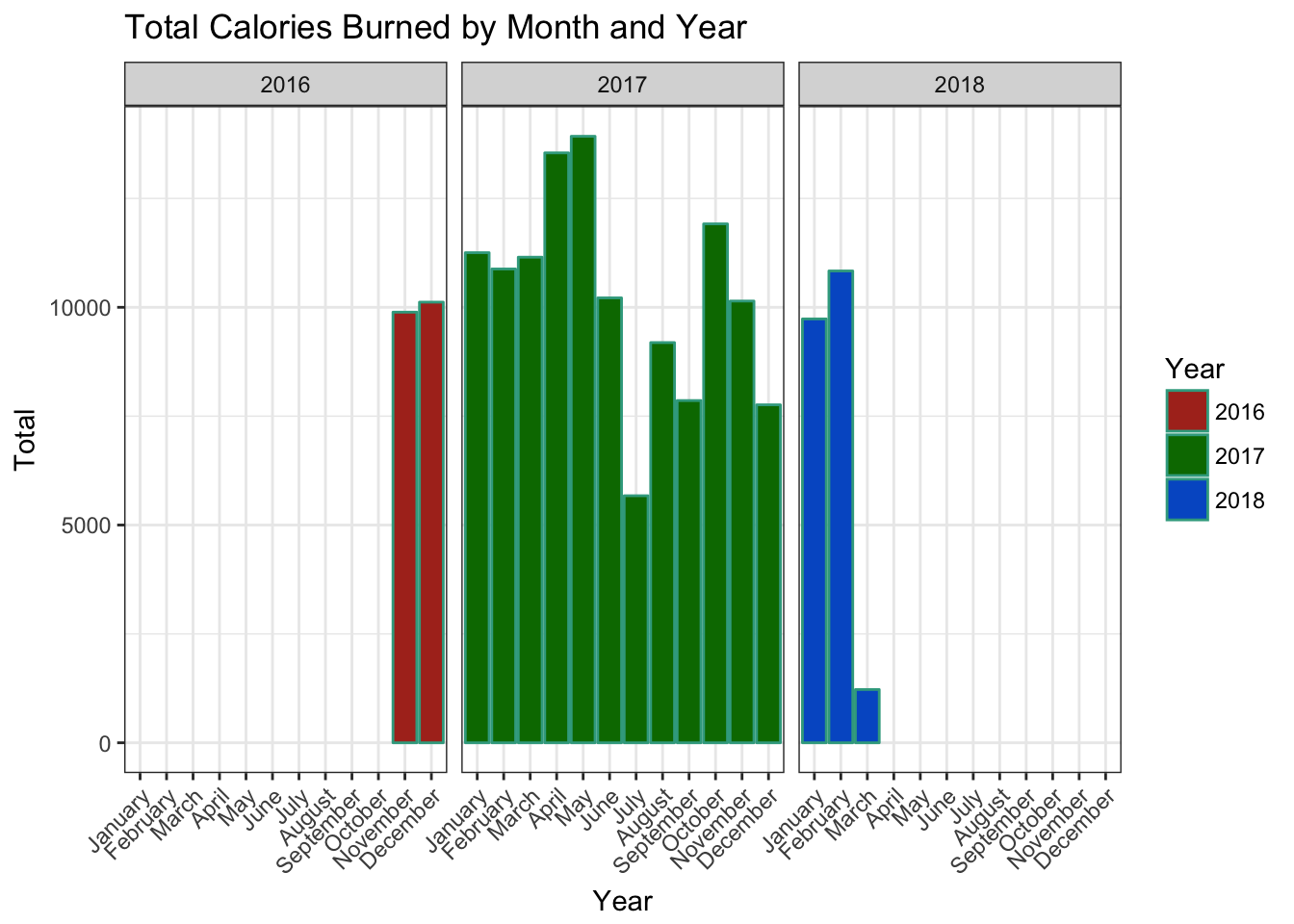I decided to only analyze the data from 2017, since that's the year with the most complete dataset. Looking at incomplete data from other years would just skew our conclusions.

## Creating tibble for 2017

Here, we will be utlizing the `filter` function to only include data which have the year column (generated from the `creationDate` column) equal to 2017 and call it `anon_data17`.

``````anon_data17 <- anon_data %>%
filter(year == 2017)
``````

## Missing Values

Although we have data for all of 2017 (by month), I wanted to explore in more detail how much data (if any) is missing from our data frame as it relates to the creation date of the recordings. I created two vectors; the first includes all entries as dates from our data frame and the second includes all dates from 2017. I then compared these two vectors to see which dates weren't recorded by the Apple Watch.

``````year_ad17 <- unique(as.Date(anon_data17\$creationDate))
year17 <- seq(as.Date("2017-01-01"), as.Date("2017-12-31"), by = "days")
missing_values <- year17[!year17 %in% year_ad17]

missing_values
``````

### Console Output

``````##   "2017-04-01" "2017-07-24" "2017-07-25" "2017-07-26" "2017-07-27"
##   "2017-07-28" "2017-07-29" "2017-07-30" "2017-07-31" "2017-08-01"
##  "2017-08-02" "2017-08-03" "2017-08-04" "2017-12-08"
``````

You can see that the missing entries include the time interval 7/24/2017 to 08/04/2017 (12 days). Most likely, there is a human-related reason why this data is missing; it could perhaps be explained by a vacation or a lost watch. Because the data is not missing at random, we have to be wary of any conclusions we make about data in this time frame.

### Summary Statistics

Now that we have an understanding of missing data we can go ahead and start exploring. I began by looking at the distribution of the calories burned (called *value`** in the data frame) using the`summary` function.

``````summary(anon_data17[["value"]])
``````

### Console Output

``````##    Min. 1st Qu.  Median    Mean 3rd Qu.    Max.
##  0.0010  0.1180  0.2080  0.5017  0.3500 11.2990
``````

This gives us great insight into some summary statistics. But visualizing data can provide insights that can't be deduced from summary statistics — so let's visualize the distribution utilizing a histogram.

``````ggplot(anon_data17,
aes(value)) +
geom_histogram(fill = "#39a78e",
bins = 200) +
theme_minimal() +
scale_x_continuous(breaks = seq(0,
max(anon_data17\$value),
by = 1)) +
labs(x = "Calories Burned",
y = "Total",
title = "Energy Burned by Month")
``````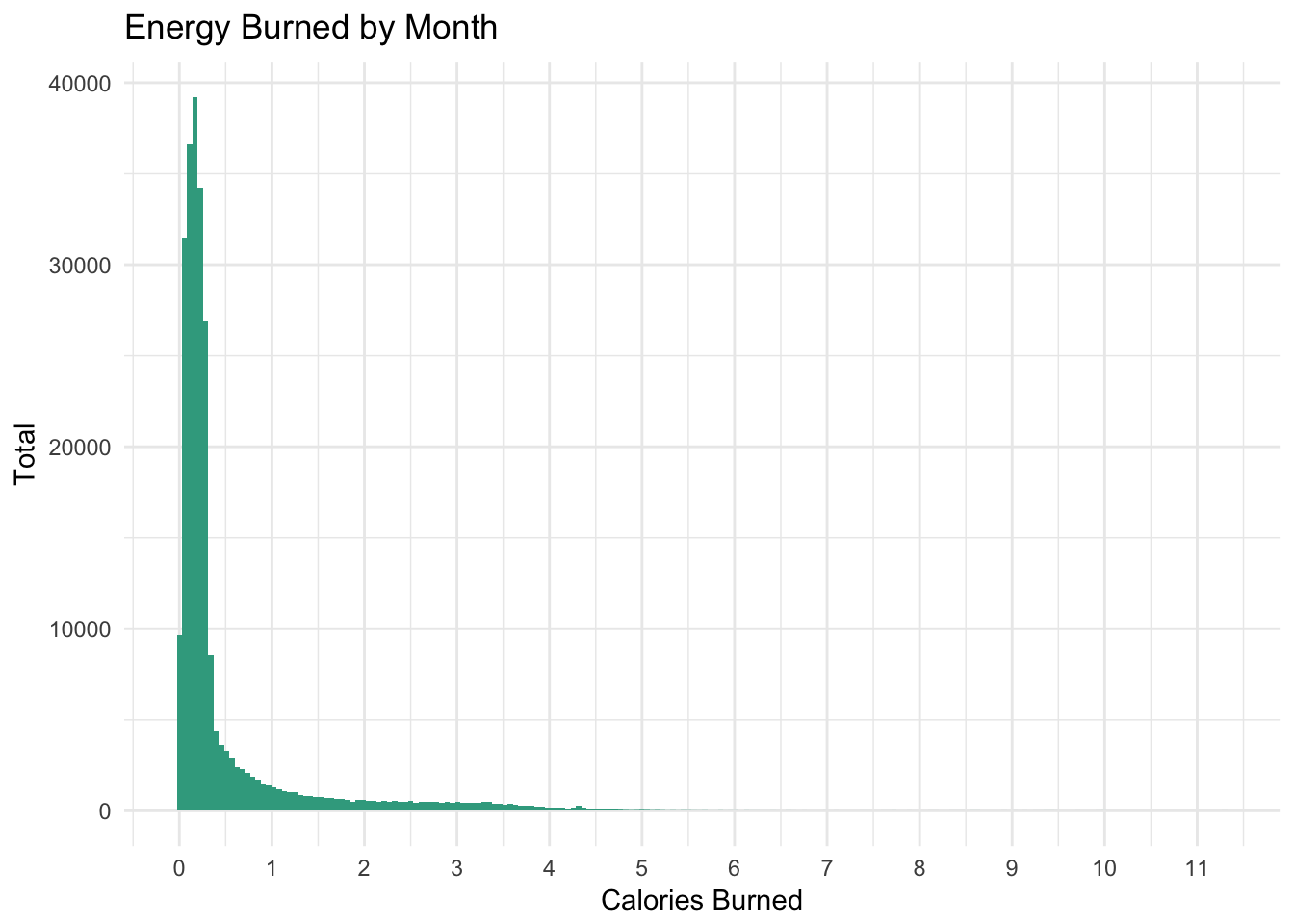We can see that there is some right skewness and that most of our data falls in the range between [0, 1].

## Data Collection by Day

I wanted to briefly look at data collection by day, so I grouped the data by creation date and counted the units and provided summary statistics.

``````anon_data17 %>%
group_by(creation_date = as.Date(creationDate)) %>%
count(unit) %>%
ungroup() %>%
select(n) %>%
summary()
# anon_data17 %>%
#   group_by(creation_date = as.Date(creationDate)) %>%
#   count(unit) %>%
#   filter(n > 900) %>%
#   arrange(desc(n))
``````

### Console Output

``````##        n
##  Min.   :   1.0
##  1st Qu.: 569.0
##  Median : 719.0
##  Mean   : 701.5
##  3rd Qu.: 855.0
##  Max.   :1276.0
``````

Here, we can see the average and median fall around 700 instances per day.

## Calendar Heatmap

Inspired by this blog post, I created a calendar heatmap to showcase the total amount of calories burned across 2017 by day of the week.

``````anon_data17 %>%
mutate(week_date = ceiling(day(creationDate) / 7)) %>%
group_by(week_date, months, week_days) %>%
summarise(total_cal = sum(value)) %>%
ggplot(.,
aes(week_days, week_date, fill = total_cal)) +
geom_tile(colour = "white") +
facet_wrap(~months) +
theme_bw() +
scale_fill_gradient(name = "Total \nCalories",
low = "#39a78e", high = "#a73952") +
labs(x = "Week of the Month",
y = "Weekday") +
scale_y_continuous(trans = "reverse")
``````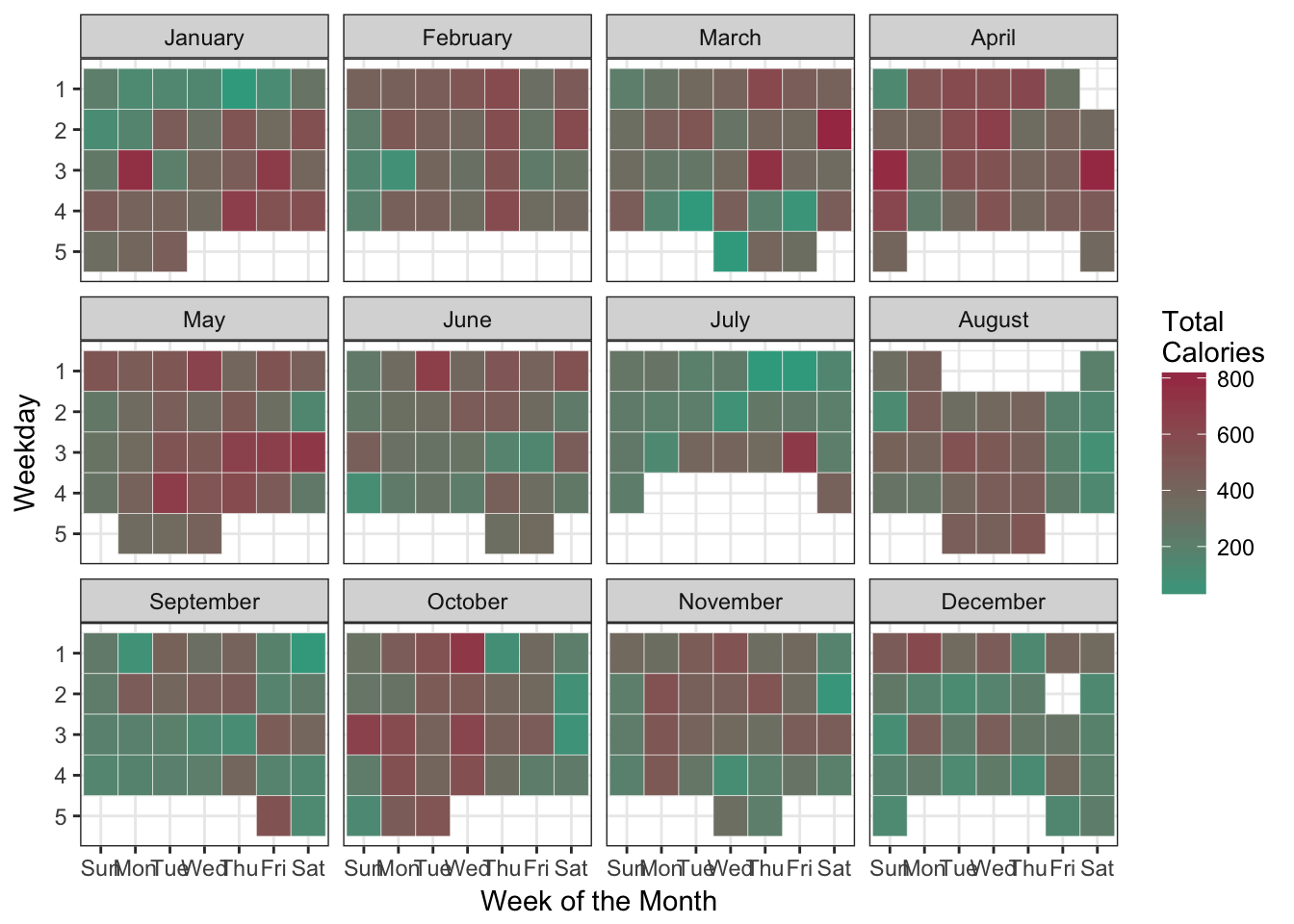## Calories and Time

Next, I wanted to shift my focus to a column I created during the data cleaning process: the time difference. I decided to explore the relationship between time range and the amount of calories burned.

``````ggplot(anon_data17,
aes(time_diff_mins, value)) +
geom_point(alpha = 0.10,
fill = "#39a78e") +
theme_minimal() +
labs(x = "Time Interval (in mins.)",
y = "Calories Burned")
``````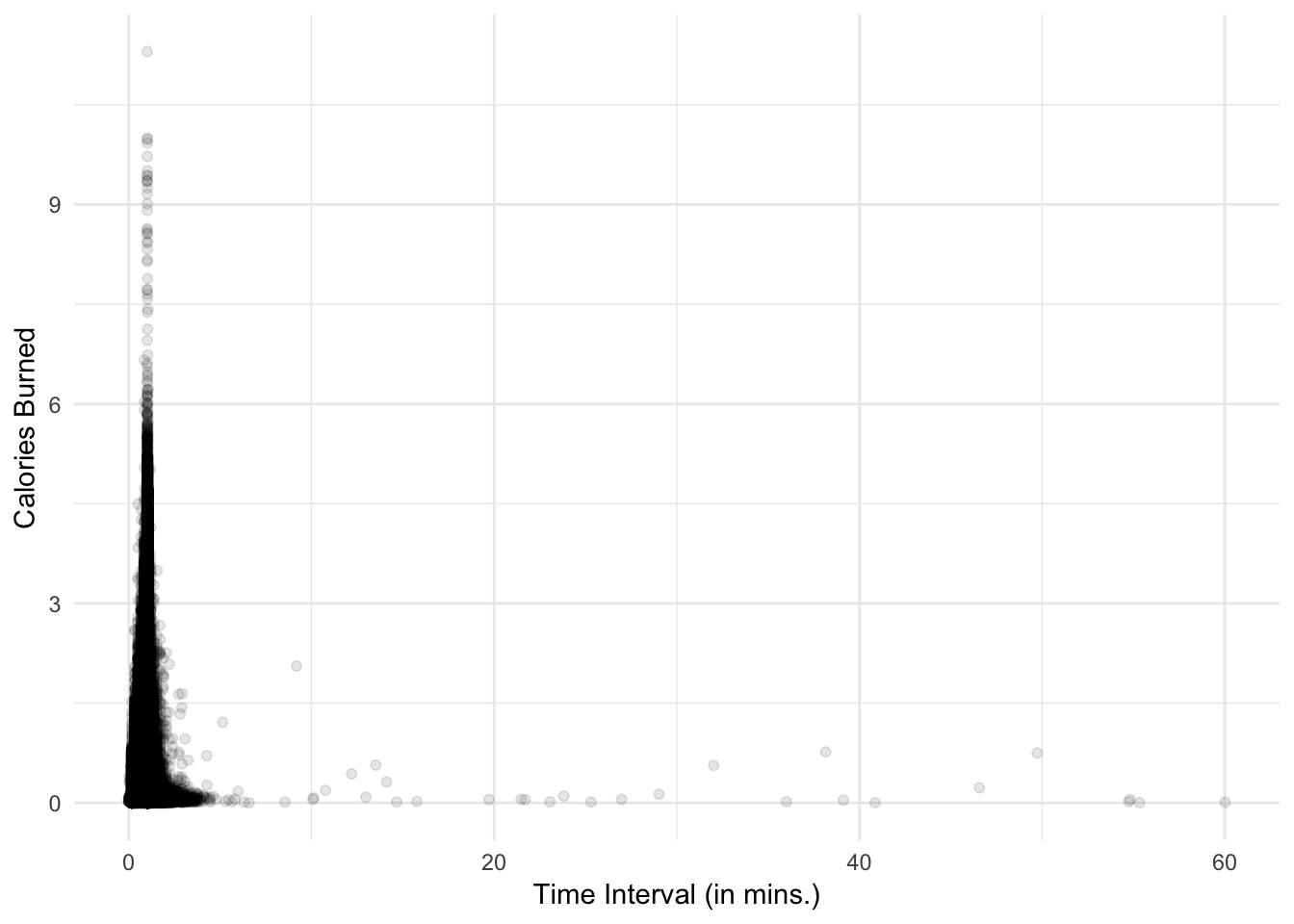Since the data is heavily skewed, this visual doesn't give us too much insight. I proceeded to log transform both variables in hopes of understanding their relationship better.

``````ggplot(anon_data17,
aes(time_diff_mins, value)) +
geom_point(alpha = 0.10,
fill = "#39a78e") +
theme_minimal() +
labs(x = "Time Interval in mins. (log transformation)",
y = "Calories Burned (log transformation)") +
scale_y_log10() +
scale_x_log10()
``````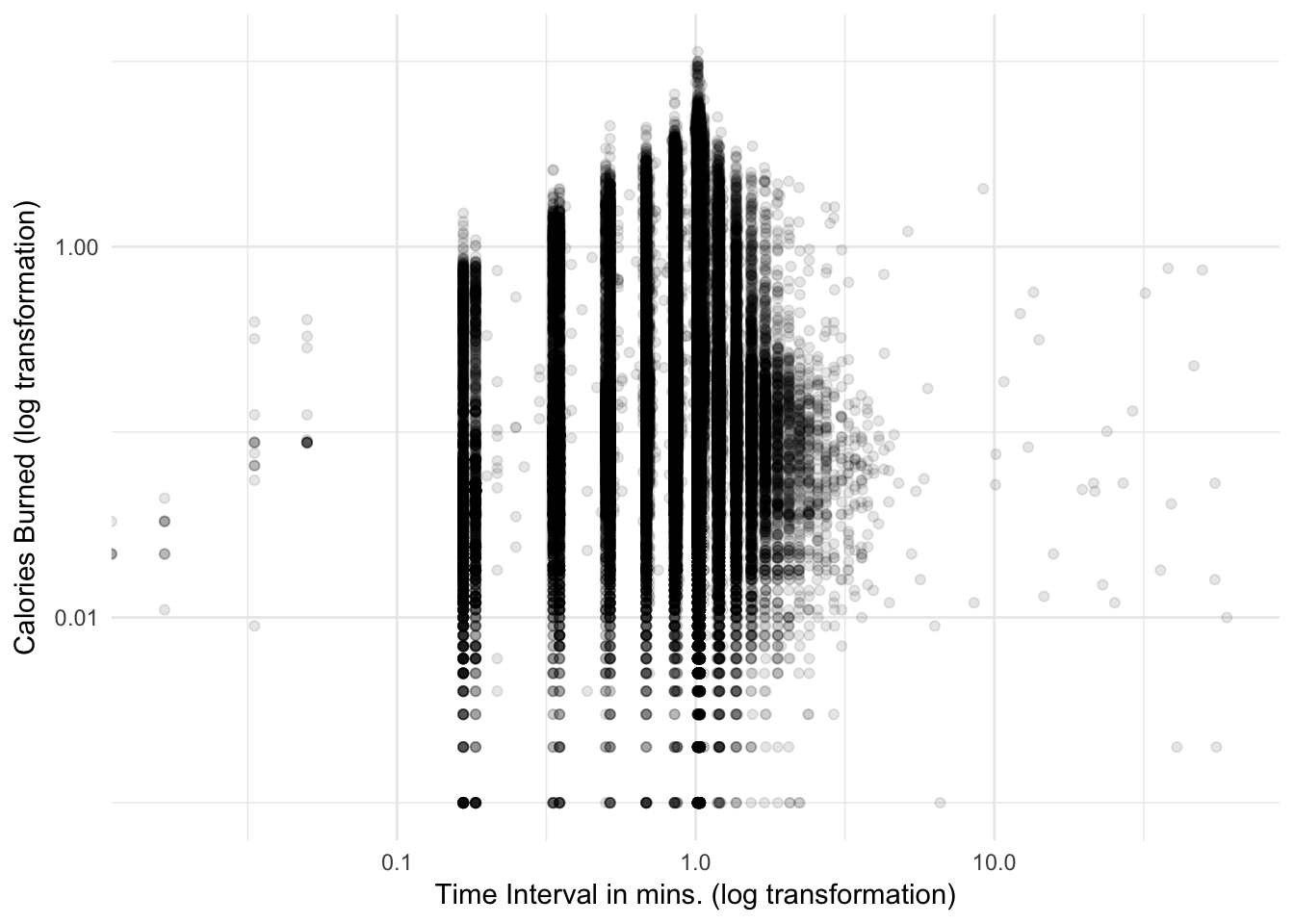Some key takeaways:

• Data capturing is mostly done in uniform time intervals
• Calories burned show an increase until around the range between [2.5, 3] minutes, at which point, the calories burned start to show a decrease
• Larger time intervals become more sporadic with respect to calories burned

## Time Frame Analysis

Next, I concentrated on the time intervals, since I found some interesting relationships between time and calories. I began by using summary on the time difference.

``````summary(anon_data17[["time_diff_mins"]])
``````

### Console Output

``````##    Min. 1st Qu.  Median    Mean 3rd Qu.    Max.
##  0.0000  1.0167  1.0167  0.9878  1.0333 60.0167
``````

You can see that the data falls mostly within the one-minute time frame and the maximum value is an hour. Let's dissect the data more by grouping both by month and by day of the week to see if there are any trends.

Let's start with grouping by month.

``````anon_data17 %>%
group_by(months) %>%
summarise(avg_time_spent = mean(time_diff_mins))
``````

### Console Output

``````## # A tibble: 12 x 2
##    months    avg_time_spent
##    <ord>              <dbl>
##  1 January            1.01
##  2 February           1.01
##  3 March              1.00
##  4 April              1.01
##  5 May                1.00
##  6 June               0.996
##  7 July               1.01
##  8 August             0.995
##  9 September          1.00
## 10 October            0.940
## 11 November           0.945
## 12 December           0.961
``````

The average for each month still gravitates around one minute. Now, let's try grouping by weekday and month. This time, since the output would be too large to read easily, I created a visual.

``````anon_data17 %>%
group_by(week_days, months) %>%
summarise(avg_time_spent = mean(time_diff_mins)) %>%
ggplot(.,
aes(week_days, avg_time_spent,
fill = week_days)) +
geom_bar(stat="identity") +
facet_wrap(~months) +
theme_bw() +
theme(axis.text.x = element_text(angle = 45,
hjust = 1)) +
labs(x = "Weekday",
y = "Average Time Spent",
title = "Average Time by months and Weekday") +
scale_fill_hue(l = 40,
name = "Weekday")
``````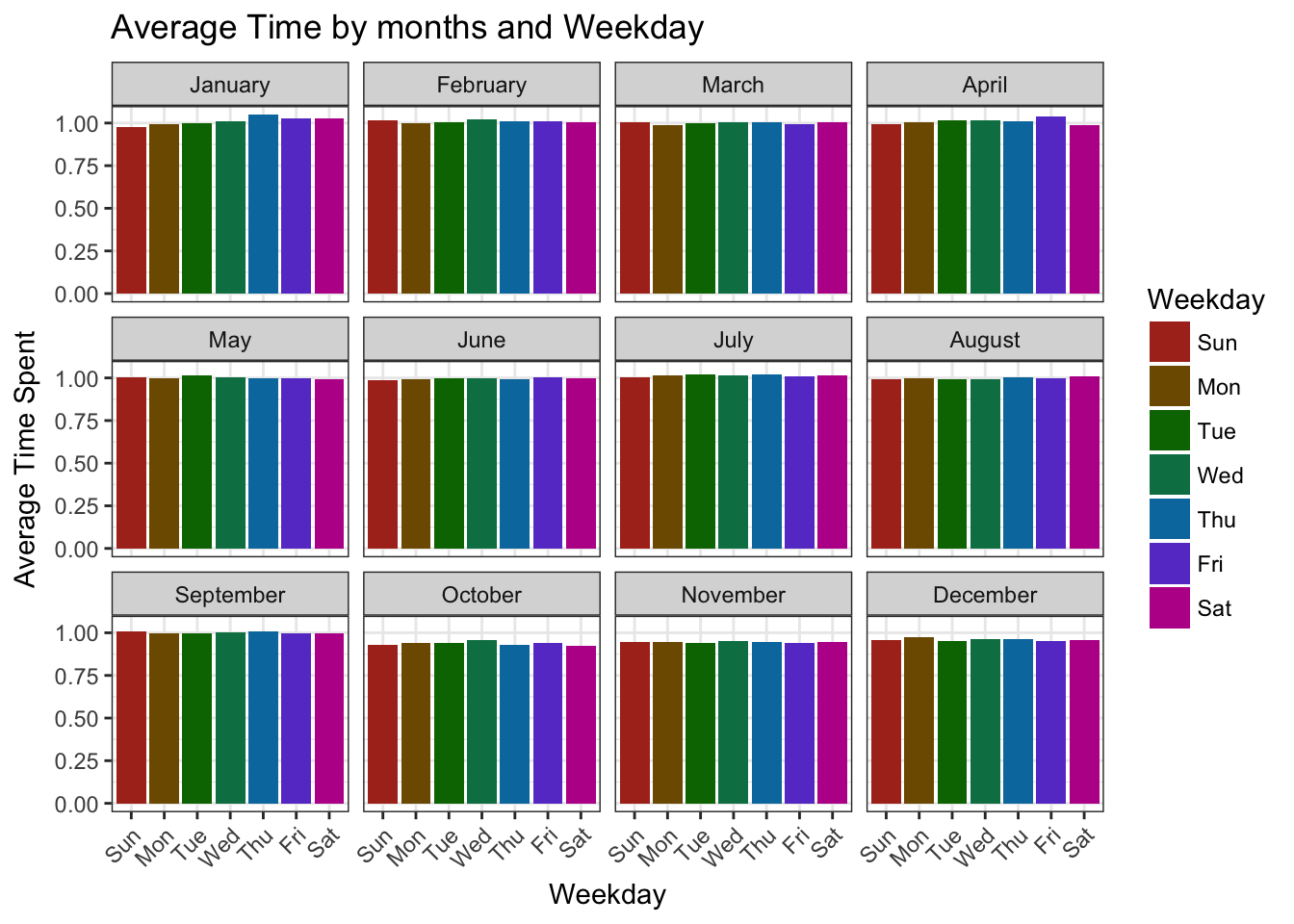Again, most of our data is collected in one-minute intervals, and there aren't any months or weekdays that show irregularities in time intervals for data collection.

Outliers are difficult to understand without knowing the specs for the Apple Watch. I'd need to do a bit more research to understand the data capturing process.

### Diving into the Time Difference Column

Let's look at time frames that are larger than 30 minutes.

``````anon_data17 %>%
filter((time_diff_mins > 30) ) %>%
select(value, time_diff_mins) %>%
arrange(desc(value))
``````

### Console Output

``````## # A tibble: 11 x 2
##    value time_diff_mins
##    <dbl>          <dbl>
##  1 0.766           38.2
##  2 0.75            49.7
##  3 0.562           32.0
##  4 0.228           46.6
##  5 0.053           54.8
##  6 0.041           39.1
##  7 0.018           36
##  8 0.016           54.8
##  9 0.01            60.0
## 10 0.002           55.3
## 11 0.002           40.8
``````

These values don't present any values larger than one, which is unusual. Upon reading this article, it appears there could have been problems with how the watch performs. However, it might be the case that during these large time intervals, the user simply wasn't that active.

## Most Data Capture by Hour

I wanted to conclude my dissection of time by looking at what hours have the highest instances of data capture. I did this by converting creation date to hour using `lubridate`.

``````ggplot(anon_data17,
aes(lubridate::hour(anon_data17\$creationDate))) +
geom_bar(fill = '#39a78e',
stat = 'count') +
theme_minimal() +
labs(x = 'Hour',
y = 'Total Count',
title = 'Data Capture by Hour')  +
scale_x_continuous(breaks = seq(0,
max(lubridate::hour(anon_data17\$creationDate)),
by = 1))
``````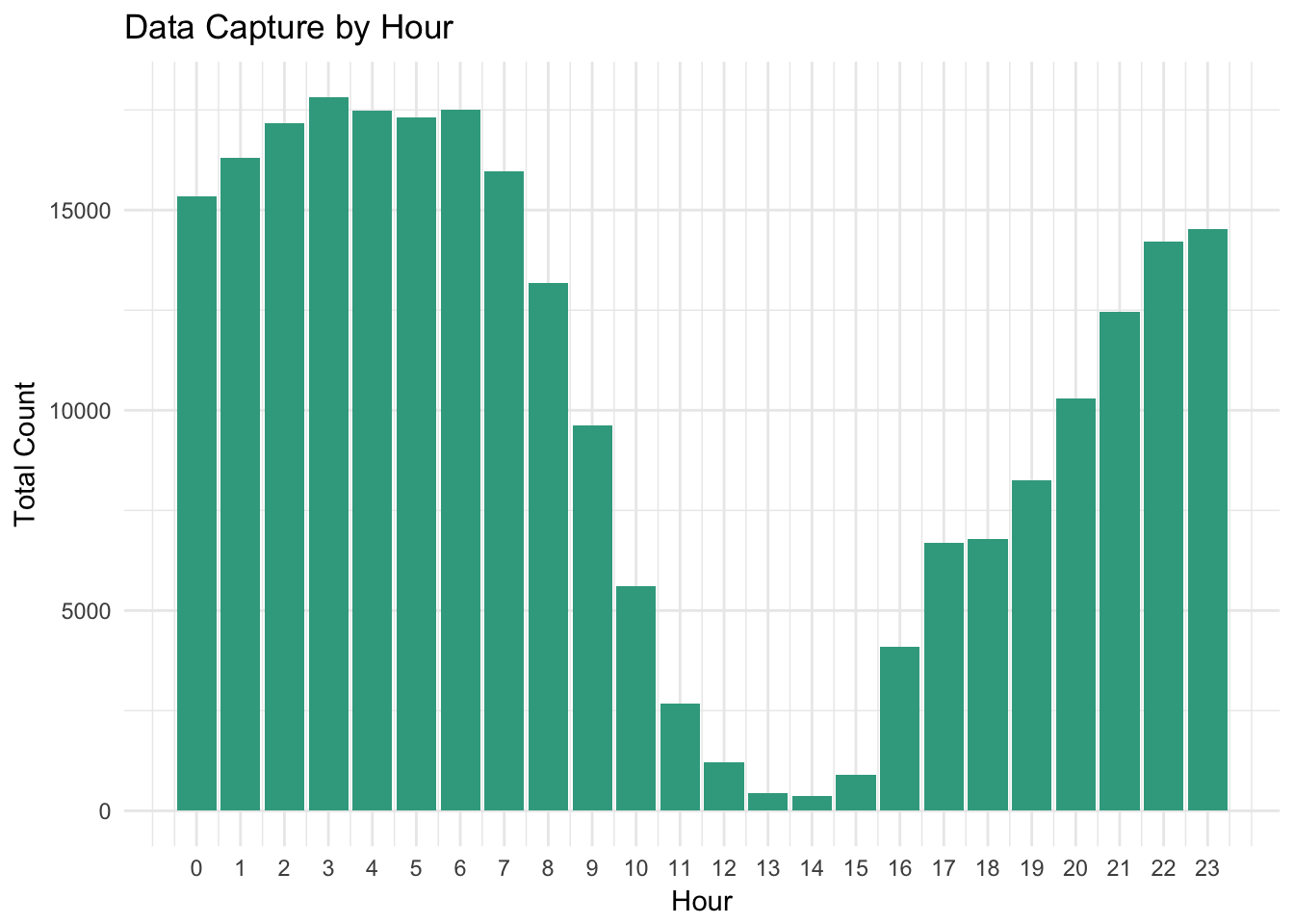You can see that data capture follows a fairly regular schedule, with a significant decrease starting at hour eight. This could be when the user is starting to go to sleep (peak hour most likely between 11 and 15).

# Calories dissected

I also wanted to visualize total calories burned by day, so I grouped the data by dates and days of the week to create a visual showing a linear view for 2017.

``````anon_data17 %>%
group_by(creation_date = as.Date(creationDate),
week_days) %>%
summarise(burn_per_day = sum(value)) %>%
ggplot(.,
aes(creation_date, burn_per_day,
fill = week_days)) +
geom_bar(stat="identity") +
theme_minimal() +
labs(x = "Creation Date",
y = "Calories burned by day",
title = "Calories by Creation Date") +
scale_fill_hue(l = 40,
name = "Weekday")
``````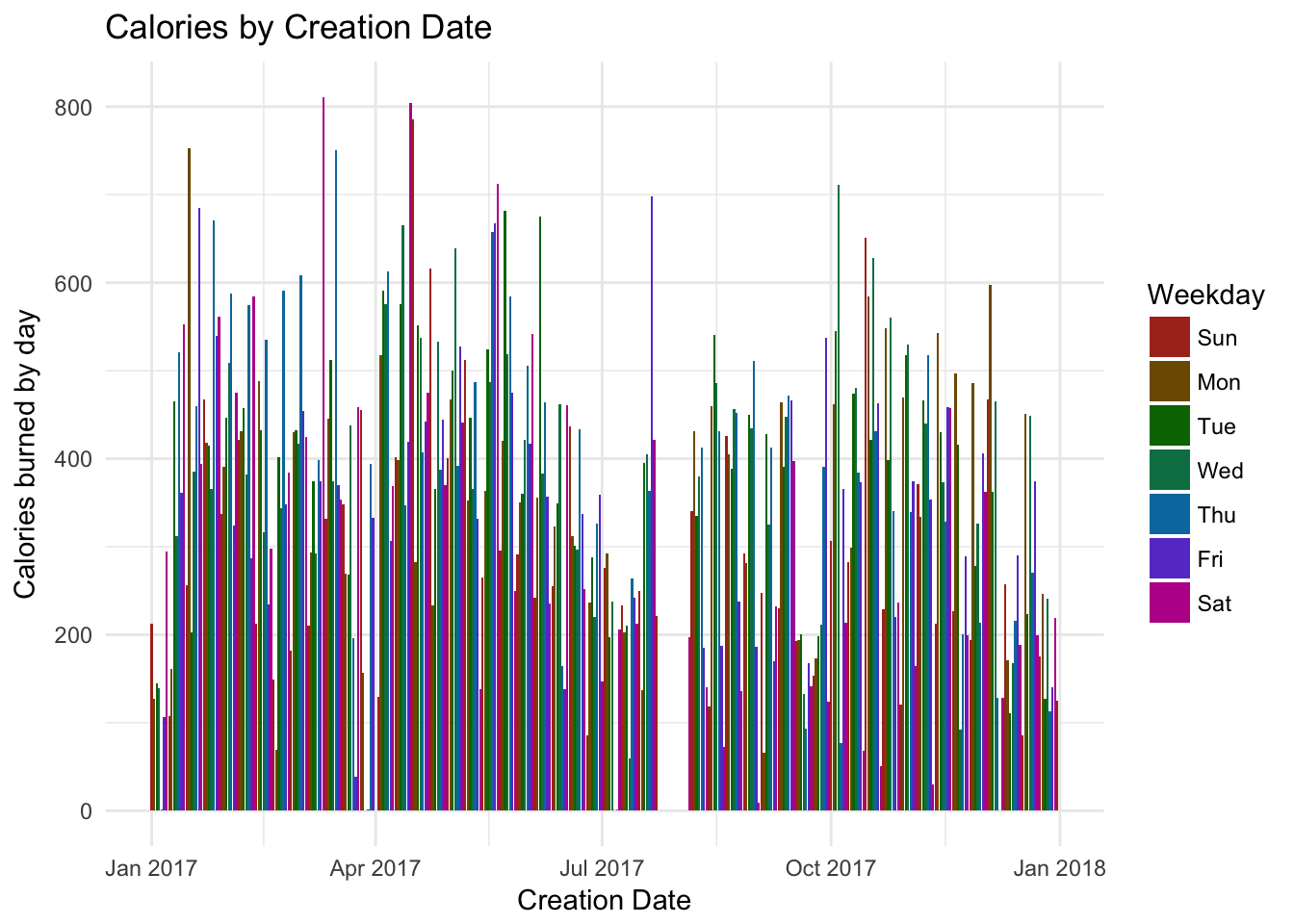As you can see, there aren't any obvious trends or seasonality, but the time frame that has missing data is easy to spot.

## Calories burned by weekday and month

Next, I went a little deeper by facet wrapping by month.

``````anon_data17 %>%
group_by(creation_date = as.Date(creationDate),
week_days, months) %>%
summarise(burn_per_day = sum(value)) %>%
ggplot(.,
aes(week_days, burn_per_day,
fill = as.factor(week_days))) +
geom_bar(stat = "identity") +
facet_wrap(~ months) +
theme_bw() +
theme(axis.text.x = element_text(angle = 45,
hjust = 1)) +
labs(x = "Weekday",
y = "Total Calories",
title = "Calories Burned by Weekday and Month") +
scale_fill_hue(l = 40,
name = "Weekday")
``````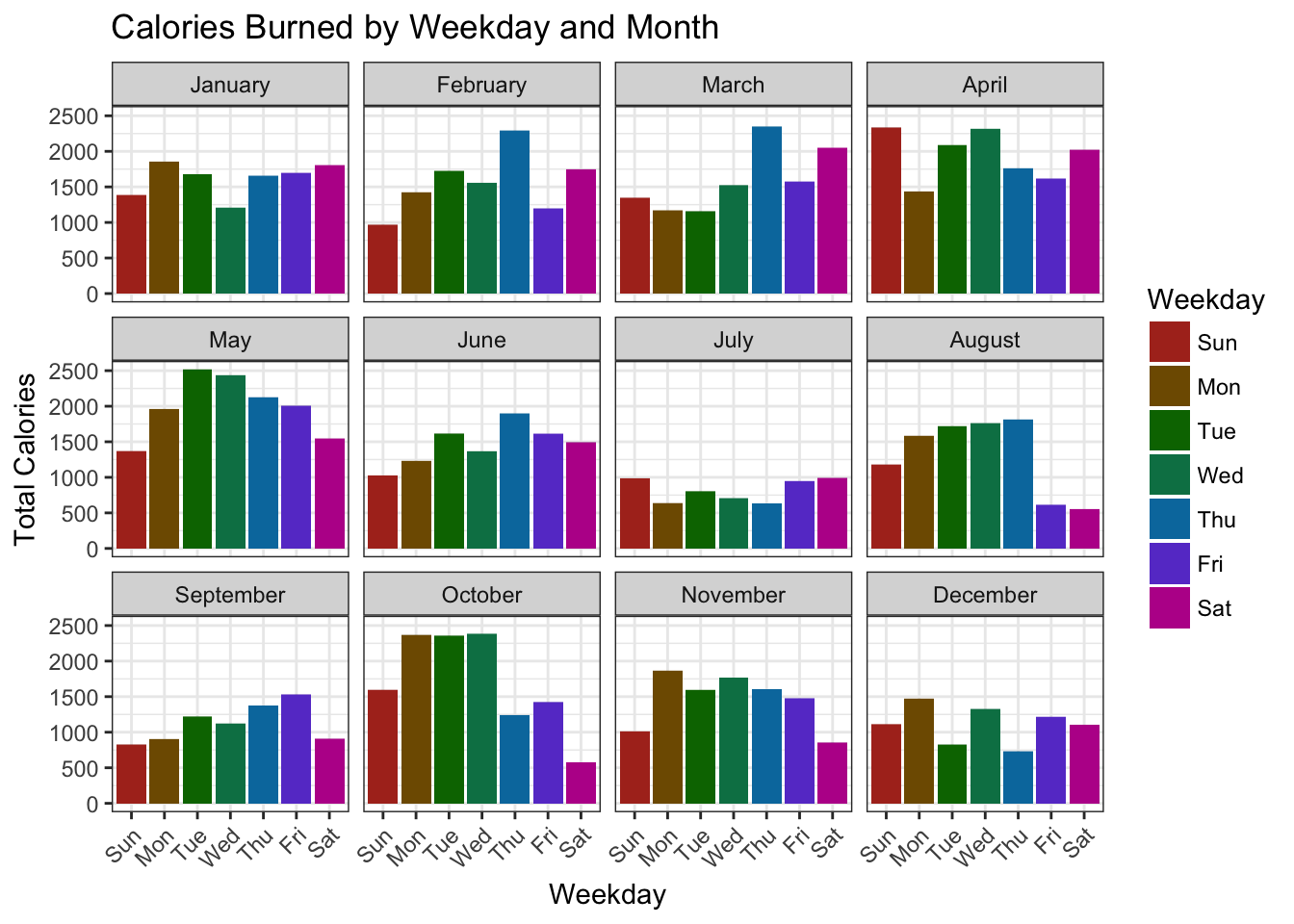You can start to see some obvious patterns — like which days of the week the user burns the most calories each month — but juxtaposing the months with days of the week would give us a better comparison.

This leads me to my next step: looking at the data from a time-series perspective. The way I did this was by averaging the values by date then plotting the results.

## Average Energy Burned for 2017

Before I visualize our data by data of the week, I wanted to visualize it as a single value by averaging energy burned by date. I accomplished this by grouping the data by start date then summarizing the calories burned by averaging. I used geom_smooth to see if there's any trend in our data set.

``````anon_data17 %>%
group_by(creation_date = as.Date(creationDate)) %>%
summarise(total_Cal = mean(value)) %>%
# filter(total_Cal == max(total_Cal))
# Used to view the date in which there was a spike in October
ggplot(.,
aes(creation_date, total_Cal)) +
geom_line(colour = "#39a78e") +
geom_smooth(se = FALSE) +
theme_minimal() +
labs(x = "Date of Activity",
y = "Average Calories",
title = "Average Energy Burned Time Series")
``````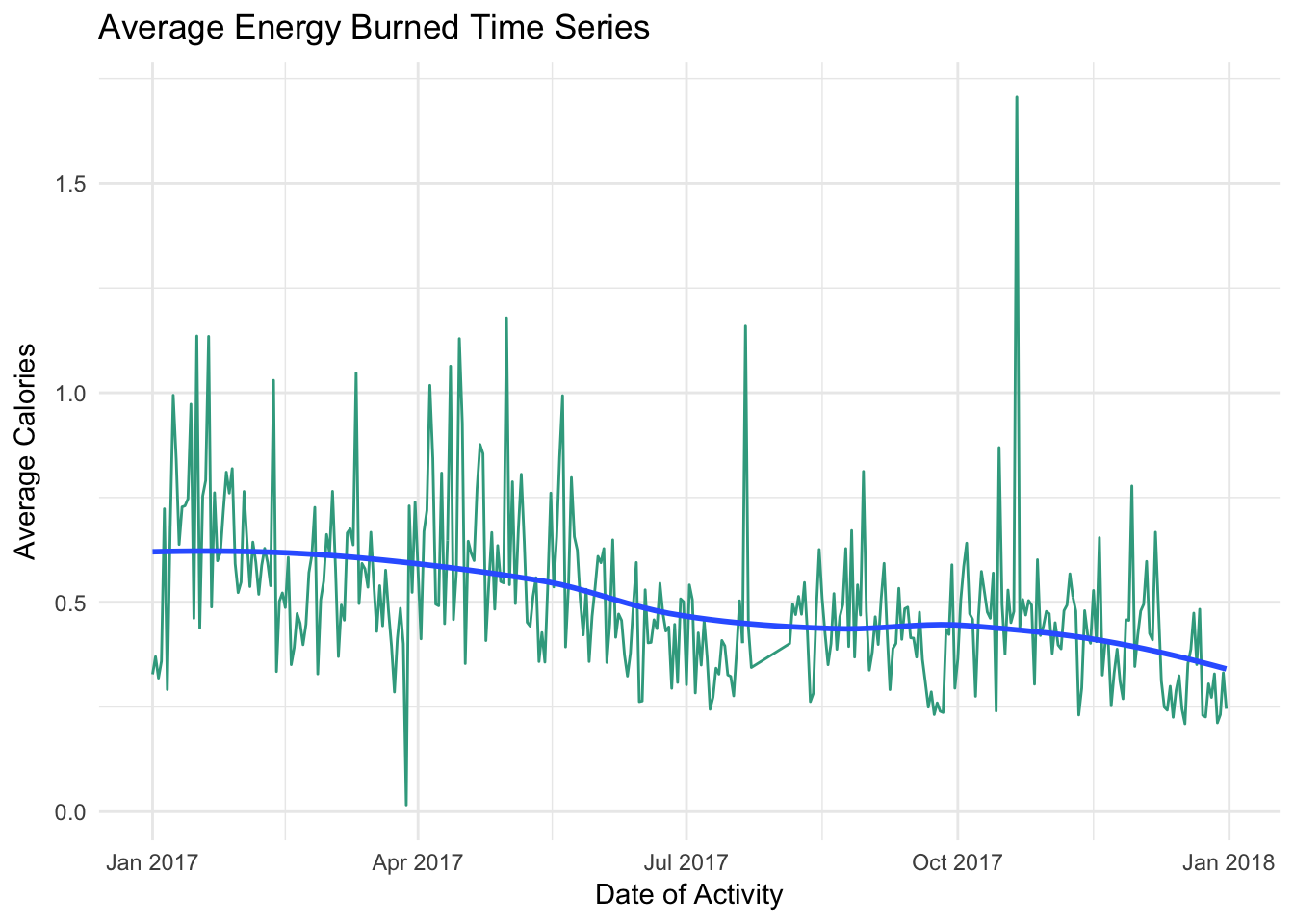We can see a slow decreasing trend with a spike in average calories on October 21, 2017. There are no obvious trends across each month.

## Average Calories by Weekday

Let's create a visual that showcases the average calories burned during the year by day of the week. This can help us understand any weekly trends that might not have been obvious in the previous plot.

``````anon_data17 %>%
group_by(week_days, year, months) %>%
summarise(total_Cal = mean(value)) %>%
ggplot(.,
aes(months, total_Cal,
group = week_days,
colour = week_days)) +
geom_line(aes(colour = week_days)) +
theme_minimal() +
labs(x = "Month",
y = "Average Calories",
title = "Average Energy Burned by Weekday for 2017") +
theme(axis.text.x = element_text(angle = 45,
hjust = 1)) +
scale_color_hue(l = 40,
name = "Weekday")
``````You might notice some seasonality in the days of the week, as well as a decreasing trend as the year goes on. This tells us that the user's activity decreased over the course of the year, which might be helpful for the user to know. With this information, we can show the user when activity decreased and help motivate them to maintain a steady level of activity.

## Conclusion

I wanted to conclude this exploratory analysis by understanding how the user burned energy throughout 2017. Most of my analysis can be applied to any data science project — hopefully it will help you extract more from your data, even if that data is not from an Apple Watch. You saw that data can be extracted from existing columns to create slices on your data frame and that visualizations provide useful information you otherwise might not glean from a table.

This project is still in its early iterations. I hope to expand it to other categories of data that the Apple Watch collects and examine the relationships across these covariates. Stay tuned!

Next Steps:

• Collect More data
• Time - Apple watch data that has more than 1 year
• Different People - Collect data from different people
• Research
• Healthly amount of calories burned daily
• Relationship between categories
• Shiny Dashboard
• Create an interactive Dashboard to showcase important visuals and summary statistics for users
• Optional choosing to allow insight into the different categories
• Machine Learning - predictive modeling
• Temporal modeling
• Creating and understanding the relationships across categories
• Data Storage
• SQL database